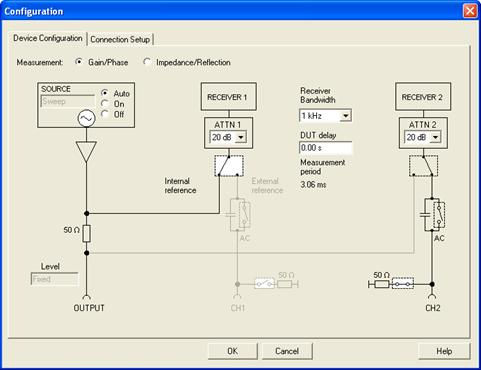top of page
Search

# FAQ #20: 2 Port Shunt Thru Impedance Measurement With The Bode 100

2 Port Shunt Thru Impedance Measurement With The Bode 100

Below are a few diagrams on how to do a 2-port impedance measurement and have the “Mag” format of the gain trace read in true Ohms. You can then choose a point on the curve that has a slope of +1 (this representing the impedance of an inductor) and using L=Z/(2*pi*f) you can accurately measure inductance as well.

Our connection diagram and 1 Ohm calibrator are shown below:Schematically this would look like:First, you would choose “Frequency Sweep”, then in the Device Configuration in the top bar of the software make sure it is set to “Gain/Phase”, have Ch1 connected to the internal reference, and terminate Ch2 into 50 Ohms.Perform a Thru calibration on both the User Calibration and Probe Calibration.You would then perform your logarithmic sweep and read Trace 1 on a logarithmic scale (for this sample 2mOhm resistor I measured 1kHz to 40MHz):This 2mOhm resistor measured slightly low at 1.8mOhms (information on why this resistor measures lower than expected will be published in a magazine article on July 5th in Electronic Design). We also used a J2102A Common Mode Transformer to remove the ground loop effects.Choosing a point where the slope is +1 (+20dB/dec) we can calculate the inductance of this 2mOhm resistor as well. It just so happens to be about 487pH: